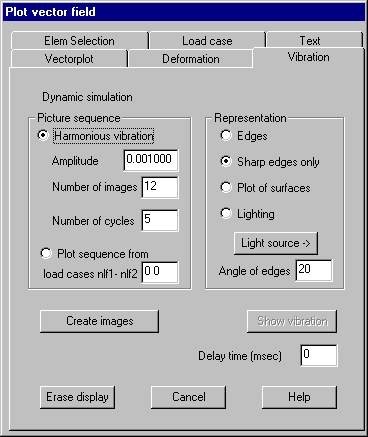Vibration: Dynamical simulation

Nodal displacements can be interpreted as amplitudes of a harmoniously vibration.

Following dialog shows the available options:Harmoniously vibration

Amplitude: The plotted length for the largest vector must be given; vectors are multiplied by this factor.

Number of images: The number of images for half the vibration cycle must be given.

Number of cycles: The number of vibration cycles to be plotted must be given.

Plot sequence from

A non harmoniously vibration can be simulated by a sequence of images. The images are created as node displacement plots defined by vector fields given in continuous load cases (for example type of input file 11). The first and last load case ID must be given.

Representation

The parameters are the same as with the command Deformation.

Create images

Clicking this button creates the sequence of images.

Show vibration

Clicking this button shows previously created images as an animation. The refresh rate heavily depends on the size of the window and the installed graphics adapter. In case of a too quick display a delay time between images (ms) can be given.

Elements: Element selection for structure respectively vector field plot

This property page allows selections for structure and vector field plot, so it’s possible to plot only vectors for selected elements. The dialog is the same as with plot of scalar fields.